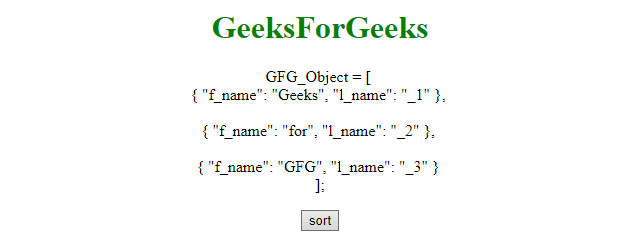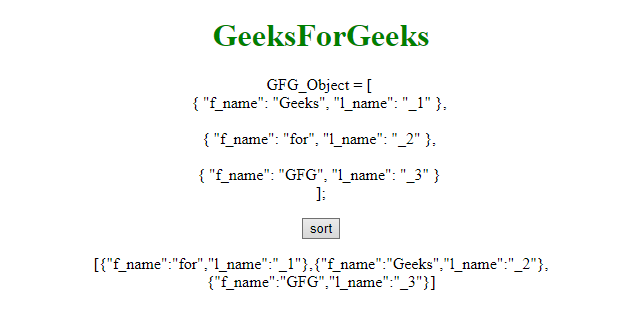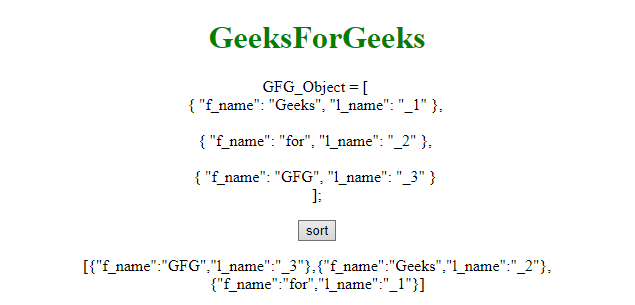# Sort array of objects by string property value in JavaScript

The array of objects can be sort by using user defined function. This function compares the array of objects by its property. For example, the first example compares the l_name of objects and if l_name is small then it place into left otherwise place it into right position.

Example 1: This example sorts the array of objects by l_name property.

 `  ` `<``html``>  ` ` `  `<``head``>  ` `    ``<``title``>  ` `        ``Sort array of objects ` `    `` ` `  ` `         `  `<``body` `style` `= ``"text-align:center;"``>  ` `     `  `    ``<``h1` `style` `= ``"color:green;"` `>  ` `        ``GeeksForGeeks  ` `    ``  ` `     `  `    ``<``p` `id``=``"demo2"``>GFG_Object = [ <``br``> ` `        ``{ "f_name": "Geeks", "l_name": "_1" }, ` `        ``<``br``><``br``> ` `        ``{ "f_name": "for", "l_name": "_2" }, ` `        ``<``br``><``br``> ` `        ``{ "f_name": "GFG", "l_name": "_3" } ` `        ``<``br``>]; ` `    ``  ` `     `  `    ``<``button` `onClick``=``"fun()"``> ` `        ``sort ` `    `` ` `     `  `    ``<``p` `id``=``"GFG"``>  ` `     `  `    ``` `    ``<``script``> ` `        ``function fun() { ` `            ``function compare(a, b) { ` `                ``if (a.l_name < ``b.l_name``) ` `                    ``return -1; ` `                ``if (a.l_name > b.l_name) ` `                    ``return 1; ` `                ``return 0; ` `            ``} ` `            ``var GFG_Object = [  ` `                ``{ f_name: 'Geeks', l_name: '_2' }, ` `                ``{ f_name: 'for', l_name: '_1' }, ` `                ``{ f_name: 'GFG', l_name: '_3' } ` `            ``]; ` `             `  `            ``GFG_Object.sort(compare); ` `             `  `            ``document.getElementById("GFG").innerHTML ` `                    ``= JSON.stringify(GFG_Object); ` `        ``} ` `        ``  ` `    ``  ` `                     `

Output:

• Before click on the button:• After click on the button:Example 2: This example sorts the array of objects by f_name property.

 `  ` `<``html``>  ` ` `  `<``head``>  ` `    ``<``title``>  ` `        ``Sort array of objects ` `    `` ` `  ` `         `  `<``body` `style` `= ``"text-align:center;"``>  ` `     `  `    ``<``h1` `style` `= ``"color:green;"` `>  ` `        ``GeeksForGeeks  ` `    ``  ` `     `  `    ``<``p` `id``=``"demo2"``> GFG_Object = [ <``br``> ` `        ``{ "f_name": "Geeks", "l_name": "_1" }, ` `        ``<``br``><``br``> ` `        ``{ "f_name": "for", "l_name": "_2" }, ` `        ``<``br``><``br``> ` `        ``{ "f_name": "GFG", "l_name": "_3" } ` `        ``<``br``>]; ` `    ``  ` `     `  `    ``<``button` `onClick``=``"fun()"``> ` `        ``sort ` `    `` ` `     `  `    ``<``p` `id``=``"GFG"``>  ` ` `  `    ``<``script``> ` `        ``function fun() { ` `            ``function compare(a, b) { ` `                ``if (a.f_name < ``b.f_name``) ` `                    ``return -1; ` `                ``if (a.f_name > b.f_name) ` `                    ``return 1; ` `                ``return 0; ` `            ``} ` `            ``var GFG_Object = [  ` `                ``{ f_name: 'Geeks', l_name: '_2' }, ` `                ``{ f_name: 'for', l_name: '_1' }, ` `                ``{ f_name: 'GFG', l_name: '_3' } ` `            ``]; ` `             `  `            ``GFG_Object.sort(compare); ` `             `  `            ``document.getElementById("GFG").innerHTML ` `                    ``= JSON.stringify(GFG_Object); ` `        ``} ` `    ``  ` `  ` ` `  `                     `

Output:

• Before click on the button:• After click on the button:My Personal Notes arrow_drop_upCheck out this Author's contributed articles.

If you like GeeksforGeeks and would like to contribute, you can also write an article using contribute.geeksforgeeks.org or mail your article to contribute@geeksforgeeks.org. See your article appearing on the GeeksforGeeks main page and help other Geeks.

Please Improve this article if you find anything incorrect by clicking on the "Improve Article" button below.